## A dealer in rural area wishes to purchase a number of sewing machines. He has only Rs. 5,760 to invest and has space for at most 20 items for storage. An  electronic sewing machine cost him Rs. 360 and a  manually operated sewing machine Rs. 240. He can  sell an electronic sewing machine at a profit of Rs. 22 and a manually operated machine at a profit of Rs. 18. Assume that the electronic sewing machines  he can sell is x and that of manually operated  machines is y.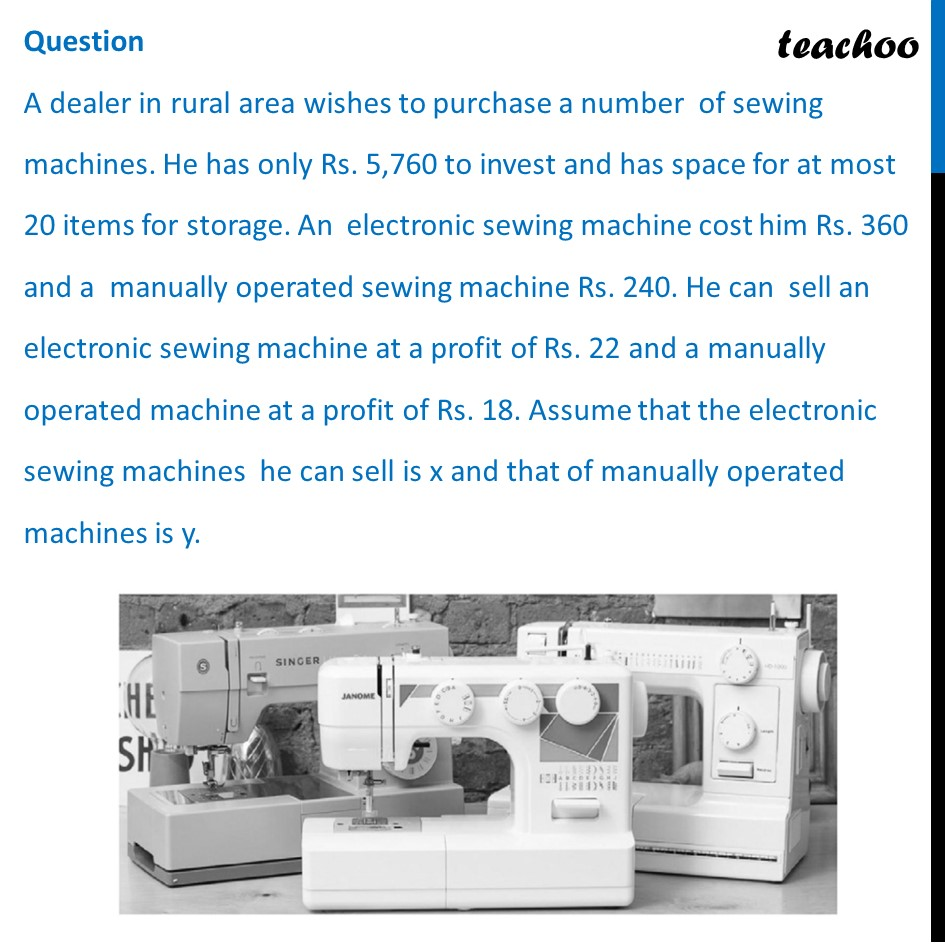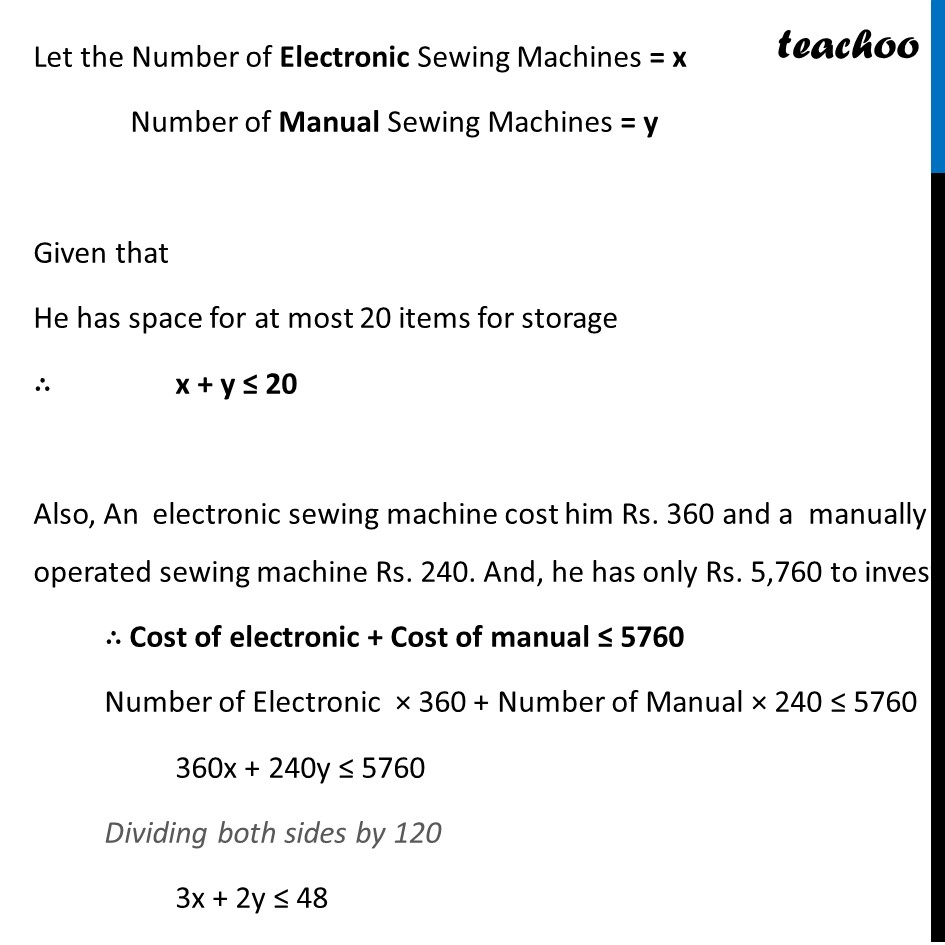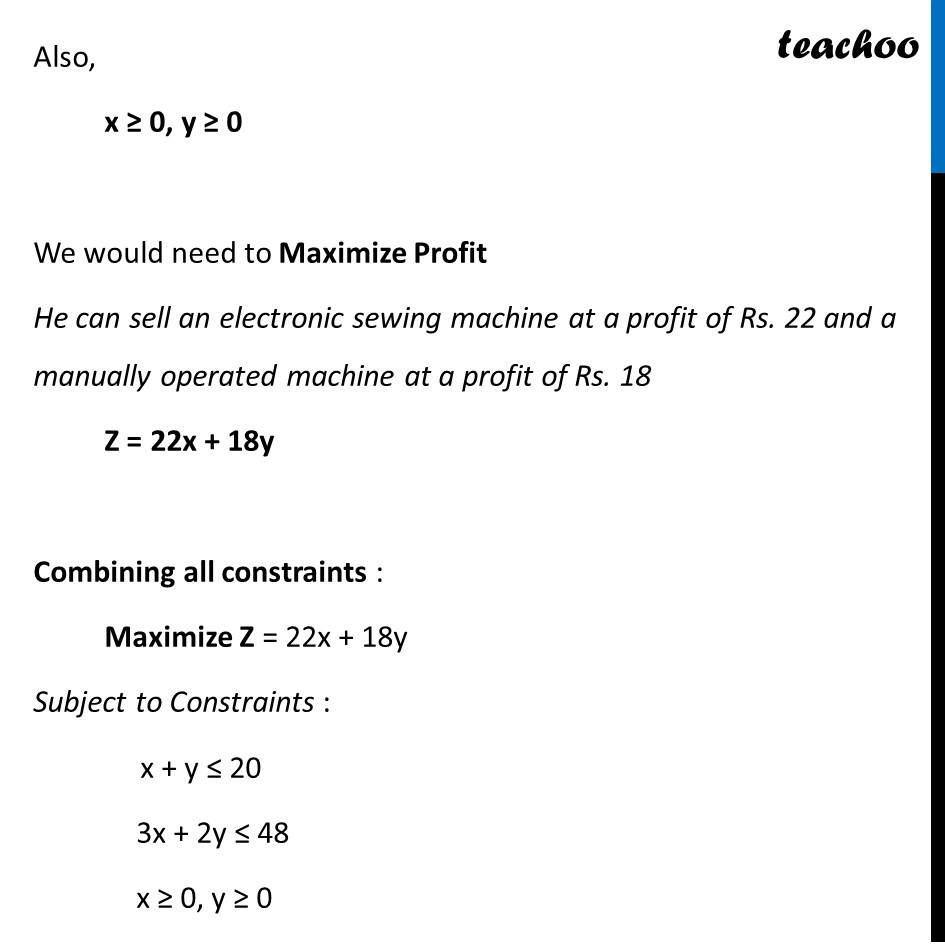## (d) Minimise Z = 22x + 18y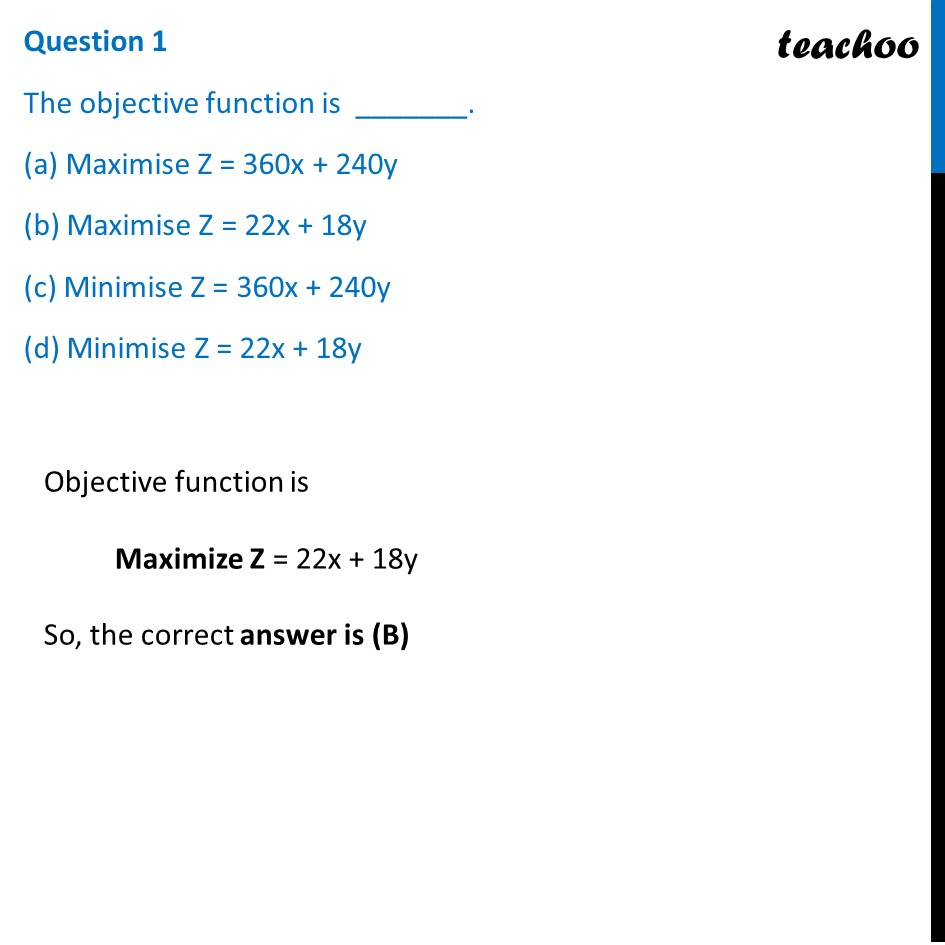## (d) 20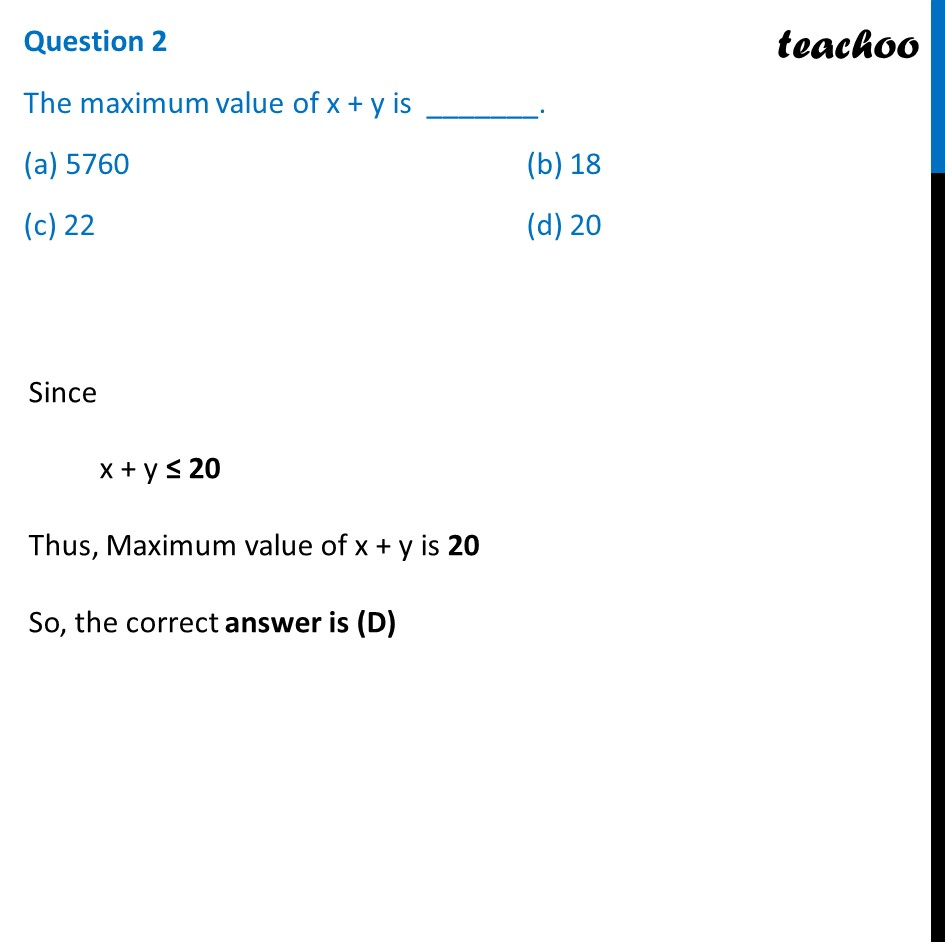## (d) y ≥ 0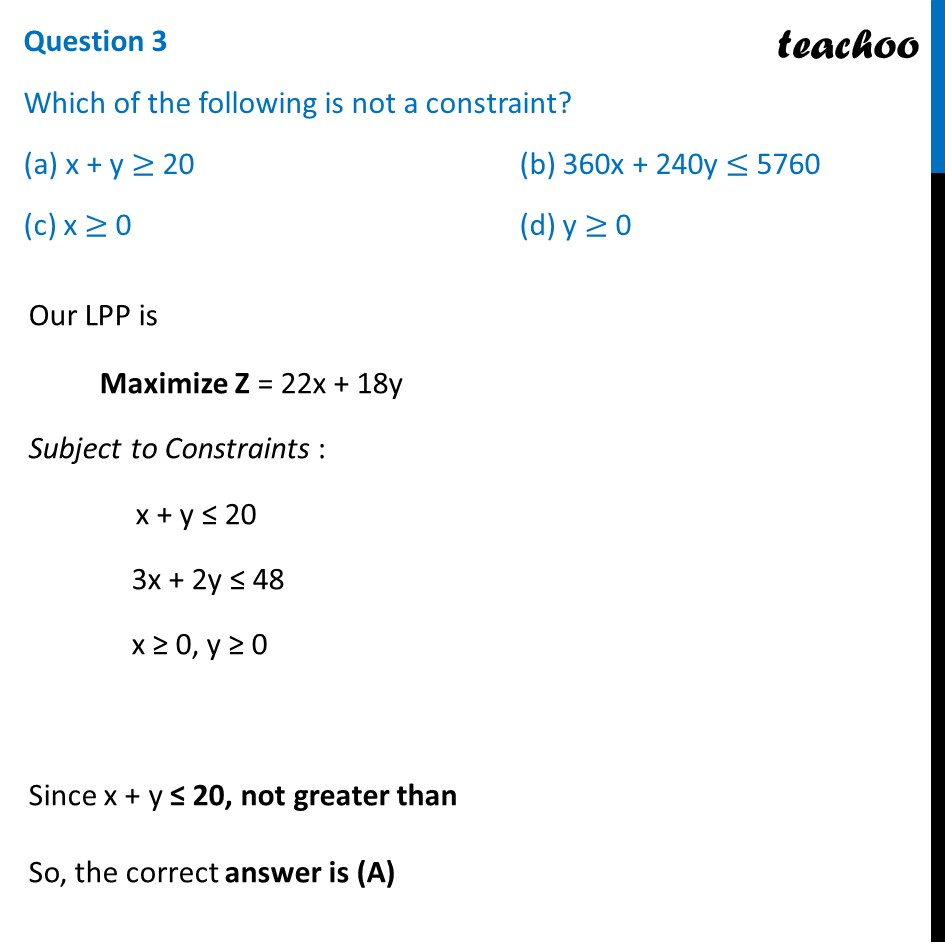## (d) (15, 5)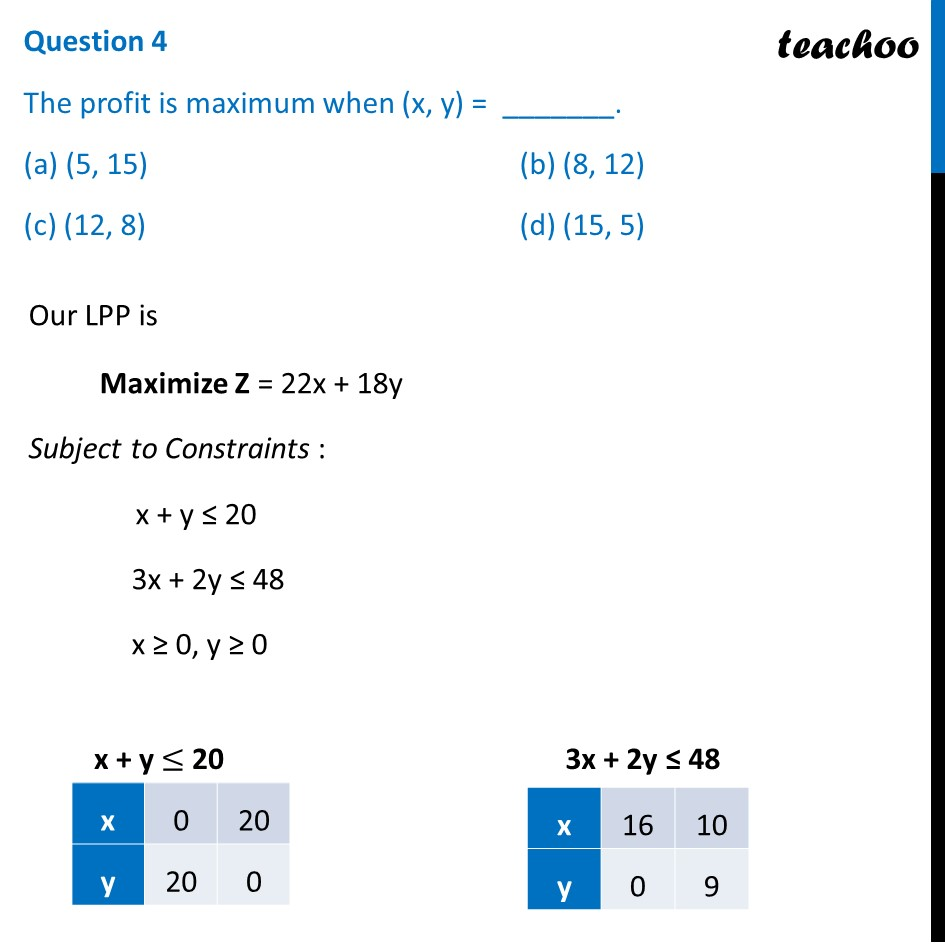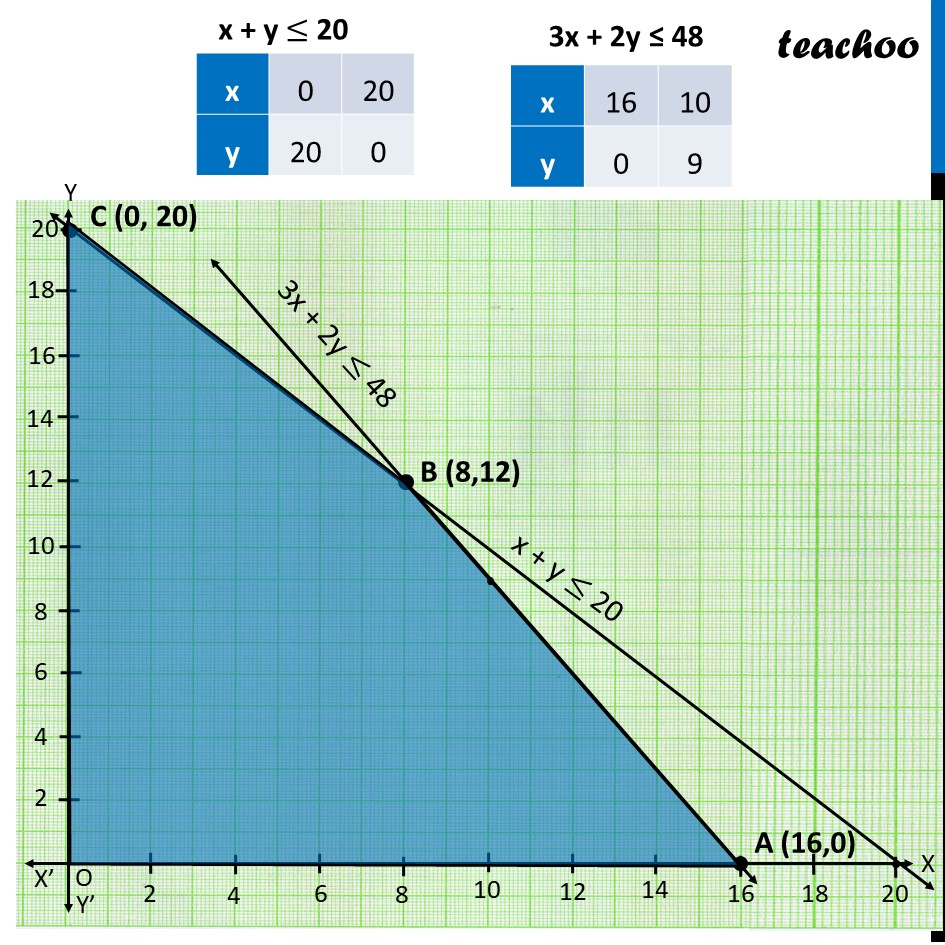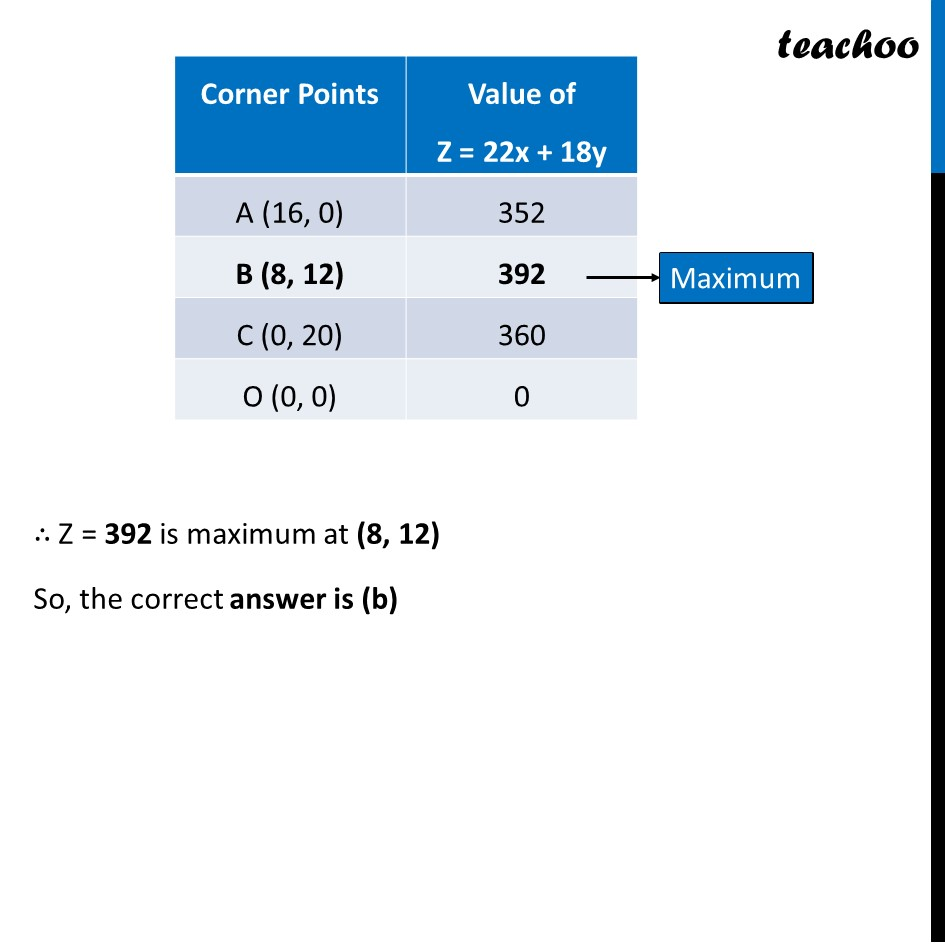## (d) 290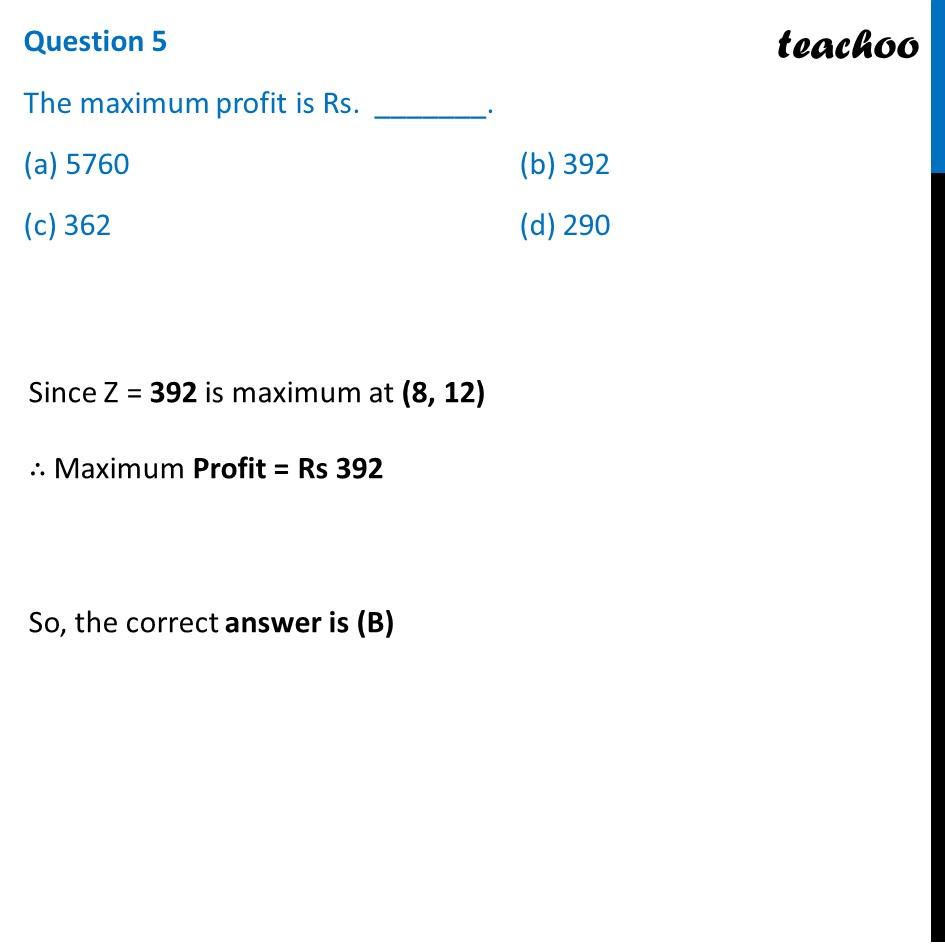1. Chapter 12 Class 12 Linear Programming (Term 1)
2. Serial order wise
3. Case Based Questions (MCQ)

Transcript

Question A dealer in rural area wishes to purchase a number of sewing machines. He has only Rs. 5,760 to invest and has space for at most 20 items for storage. An electronic sewing machine cost him Rs. 360 and a manually operated sewing machine Rs. 240. He can sell an electronic sewing machine at a profit of Rs. 22 and a manually operated machine at a profit of Rs. 18. Assume that the electronic sewing machines he can sell is x and that of manually operated machines is y. Let the Number of Electronic Sewing Machines = x Number of Manual Sewing Machines = y Given that He has space for at most 20 items for storage ∴ x + y ≤ 20 Also, An electronic sewing machine cost him Rs. 360 and a manually operated sewing machine Rs. 240. And, he has only Rs. 5,760 to invest ∴ Cost of electronic + Cost of manual ≤ 5760 Number of Electronic × 360 + Number of Manual × 240 ≤ 5760 360x + 240y ≤ 5760 Dividing both sides by 120 3x + 2y ≤ 48 Also, x ≥ 0, y ≥ 0 We would need to Maximize Profit He can sell an electronic sewing machine at a profit of Rs. 22 and a manually operated machine at a profit of Rs. 18 Z = 22x + 18y Combining all constraints : Maximize Z = 22x + 18y Subject to Constraints : x + y ≤ 20 3x + 2y ≤ 48 x ≥ 0, y ≥ 0 Question 1 The objective function is _______. (a) Maximise Z = 360x + 240y (b) Maximise Z = 22x + 18y (c) Minimise Z = 360x + 240y (d) Minimise Z = 22x + 18y Objective function is Maximize Z = 22x + 18y So, the correct answer is (B) Question 2 The maximum value of x + y is _______. (a) 5760 (b) 18 (c) 22 (d) 20 Since x + y ≤ 20 Thus, Maximum value of x + y is 20 So, the correct answer is (D) Question 3 Which of the following is not a constraint? (a) x + y ≥ 20 (b) 360x + 240y ≤ 5760 (c) x ≥ 0 (d) y ≥ 0 Our LPP is Maximize Z = 22x + 18y Subject to Constraints : x + y ≤ 20 3x + 2y ≤ 48 x ≥ 0, y ≥ 0 Since x + y ≤ 20, not greater than So, the correct answer is (A) Question 4 The profit is maximum when (x, y) = _______. (a) (5, 15) (b) (8, 12) (c) (12, 8) (d) (15, 5) Our LPP is Maximize Z = 22x + 18y Subject to Constraints : x + y ≤ 20 3x + 2y ≤ 48 x ≥ 0, y ≥ 0 So, the correct answer is (B) Question 4 The profit is maximum when (x, y) = _______. (a) (5, 15) (b) (8, 12) (c) (12, 8) (d) (15, 5) Our LPP is Maximize Z = 22x + 18y Subject to Constraints : x + y ≤ 20 3x + 2y ≤ 48 x ≥ 0, y ≥ 0 ∴ Z = 392 is maximum at (8, 12) So, the correct answer is (b) Question 5 The maximum profit is Rs. _______. (a) 5760 (b) 392 (c) 362 (d) 290 Since Z = 392 is maximum at (8, 12) ∴ Maximum Profit = Rs 392 So, the correct answer is (B)

Case Based Questions (MCQ)